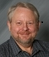## Reasonable SQL queryTags:
SQL
SQL Query
Do you this this is a reasonable SQL query to have in an application?

`sSQL = "select distinct "; sSQL += " S.ChainID,S.State,S.City,S.ChainCode,S.StoreNumber,"; sSQL += " DD.DeptID,D.DeptNum,DD.DeptDesc,DD.ReportGrpid ,' ' as tax1,' ' as tax2,' ' as tax3,DiscID "; sSQL += " ,convert(varchar(10),DiscStartDate) as DiscStartDate, convert(varchar(5),DiscStartTime) as DiscStartTime ,"; sSQL += " convert(varchar(10),DiscEndDate) as DiscEndDate,convert(varchar(5),DiscEndTime) as DiscEndTime , PricePoint,"; sSQL += " convert(varchar(3),TrivDiscID) as TrivDiscID into tmpdeptfile "; sSQL += " from Store_Info_Data S "; sSQL += " left outer join tblBrandDept BD "; sSQL += " on S.BrandID = BD.BrandID "; sSQL += " left outer join tblDepartments D "; sSQL += " on BD.DeptID = D.DeptID "; sSQL += " left outer join tblDeptDesc DD "; sSQL += " on (S.LanguageCode = DD.LanguageCode and D.DeptID = DD.DeptID )"; sSQL += " left outer join "; sSQL += " ("; sSQL += " select distinct Deptid, SDID.DiscID, SDID.ChainID, StoreNum, AutoDiscName, TrivDiscID, DiscStartDate,"; sSQL += " DiscStartTime, DiscEndDate, DiscEndTime, PricePoint "; sSQL += " from tblDeptDiscID DDID "; sSQL += " inner join tblStoreDiscID SDID "; sSQL += " on (DDID.Discid = SDID.discid) "; sSQL += " left outer join tblDiscountRules DR "; sSQL += " on (dr.Discid = sdid.discid) and DR.ChainID = SDID.ChainID "; sSQL += " ) x "; sSQL += " on D.DeptID = X.DeptID and x.ChainID = S.ChainID and x.StoreNum = S.StoreNumber "; sSQL += " WHERE S.State is not null and s.state not in ('TK Maxx') and s.ChainID is not null"; sSQL += " and D.DeptID is not null"; sSQL += " order by s.chaincode, s.storenumber, dd.DeptDesc";`

Software/Hardware used:
SQL server 2008, C#

Thanks. We'll let you know when a new response is added.

## Discuss This Question: 4 Replies

Thanks. We'll let you know when a new response is added.
••••